## FSA’s Methodology

FSA’s methodology is based on understanding a company’s ability to generate a return on invested capital and the value of cash flow accruing to its shareholders

## FSA’s Three Phase Method

• Performance Measurement
• Forecasting
• Valuation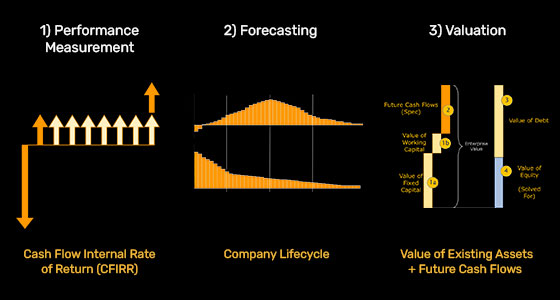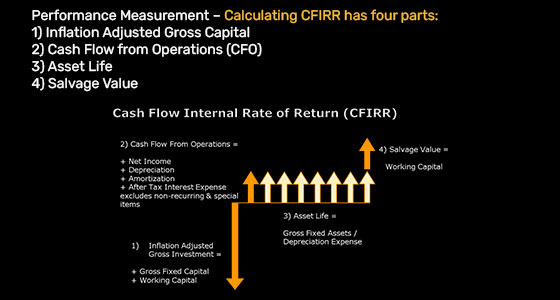## Performance Measurement

### Cash Flow Internal Rate of Return - CFIRR

The crux of our methodology is a unique performance measurement called Cash Flow Internal Rate of Return (CFIRR). CFIRR addresses many of the shortcomings of traditional performance measurements such as Return on Equity (ROE), Return on Assets (ROA) and Return on Invested Capital (ROIC). In principle, CFIRR measures the return on capital but has distinct advantages over traditional return on capital measures. Interestingly, the acronym RoC in our monthly "The RoC Report" stands for Return on Capital and was developed as a marketing term that was simpler and more effective than "The CFIRR Report." In our own reports, the term "return on capital," or simply the term "return," is used interchangeably with CFIRR. However, in studying the details of the methodology it is important to make the distinction between CFIRR and traditional return on capital measures. CFIRR should be viewed in context of capital growth and the cost of capital. We will discuss this further after we discuss how we calculate CFIRR.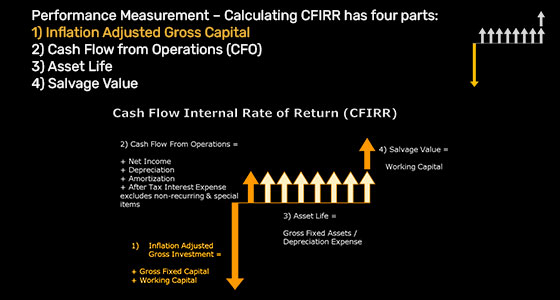Conceptually, invested capital is long-term debt + equity = assets – working capital. This definition is sufficient when conceptualizing a company's invested capital, however, there are other details to note. Finance textbooks show two methods of calculating invested capital: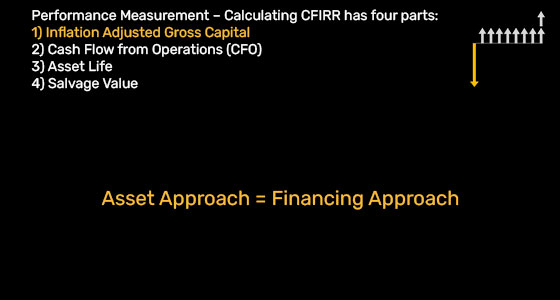### Asset Approach = Financing Approach

A) Asset Approach =
+ Short-Term Assets
- Short-Term Liabilities (excluding short-term debt)
+ Net Property, Plant and Equipment
+ Other Long-Term Assets
+ Intangibles

B) Financing Approach =
+ Common Equity
+ Preferred Stock
+ Minority Interest
+ Deferred Income Taxes
+ Long-Term Debt
+ Other Long-Term Liabilities
+Short-Term Debt

These two methods will always reconcile.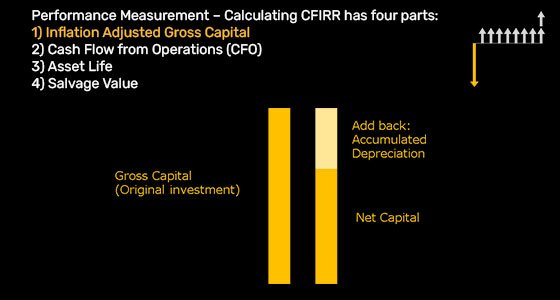### CFIRR Uses GROSS INVESTMENT

The first adjustment to invested capital is to use GROSS investment - not the NET investment. In other words, accumulated depreciation is added back to the net investment to get gross investment.

This is done to calculate the return on total original capital contributed to the business.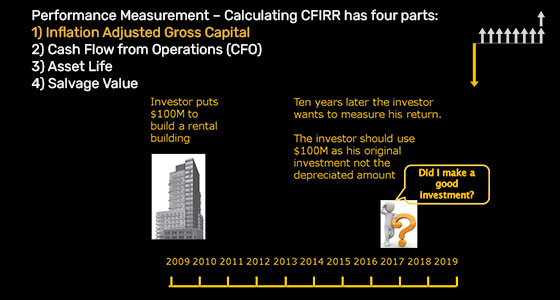### Example: Building a Condo for Rental Income

Suppose a landowner was considering building condos to be rented by the public. The landowner would determine how much up front capital would be required to build the building. Then the landowner would estimate rates, including rental and occupancy, to estimate the total cash flow going forward. He would then make a determination on whether or not to build based on the expected internal rate of return. Suppose 10 years down the road the landowner wanted to determine if the decision to build had been favourable. In post-mortem analysis, the landowner would look at actual rental rates and occupancy to calculate cash flow, and would still want to know the amount of his original investment, or gross investment. He would not ask his accountant how much the building had depreciated to calculate the return. It is not relevant. In capital budgeting projects managers and analysts estimate the return on gross investment. The return on gross investment is estimated in every finance situation. It follows that the same should be done for publicly traded companies. It is worth noting at this point that gross investment is used to determine the return on capital, but net investment will be used to determine the value of the company. (See Valuation section)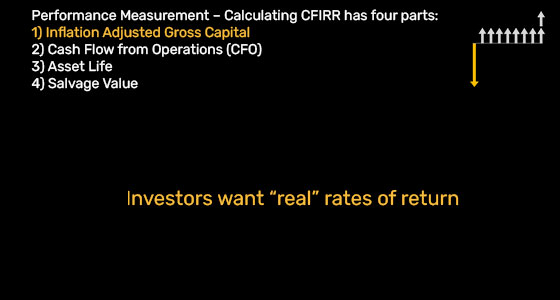Investors are interested in a “real” return not a nominal return. Since cash flow and earnings are in today's dollars, it is necessary to adjust the gross investment into today's dollars that is carried on the balance sheet at historical costs.

To clarify why this is important, consider a period of high inflation vs. low inflation. A company's cash flow from operations will be much higher during high inflationary periods compared to in low inflationary periods, but it is not attributable to real improvements in the business but rather inflation.

## Cash Flow from Operations (CFO)

Cash flow from operations is the after tax cash flow accruing to capital providers in a given year. It's the amount of money management can spend on new projects, pay dividends, etc. By contrast, you can't spend earnings, and therefore, cash flow is a better measure of value.  Moreover, earnings are more easily manipulated by accounting policy whereas cash flow is not.

Cash Flow from Operations =
+ Net Income
+ After-Tax Non-Operating Income / Expense
+ After-Tax Interest Expense
+ After-Tax Minority Interest
+ Depreciation and Amortization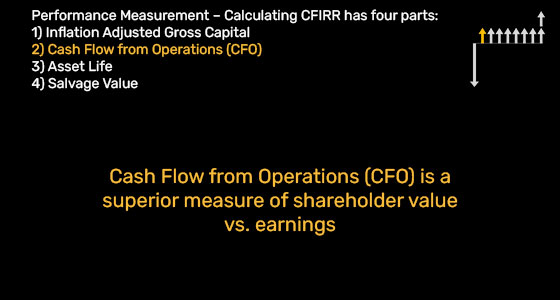### Unlevered Analysis

It should be noted that CFO is a pre-interest measure of profitability to facilitate the comparison of firms with different degrees of leverage. Using post-interest measures of profit negates this and makes leveraged firms appear less profitable. Also, since interest is tax deductible, interest payments must be tax-effected when a post-tax measure of profits is used. CFIRR adds back after-tax interest expense. In other words, unleveraged firms do not get a tax shield from interest payments. Therefore, to compare operating profitability between leveraged firms and unleveraged firms, after tax-interest should be added back to net income. It follows that adding back after-tax non-operating income is also correct. In contrast, it is correct to add back pre-tax depreciation and amortization as both leveraged and unleveraged firms would get a tax shield.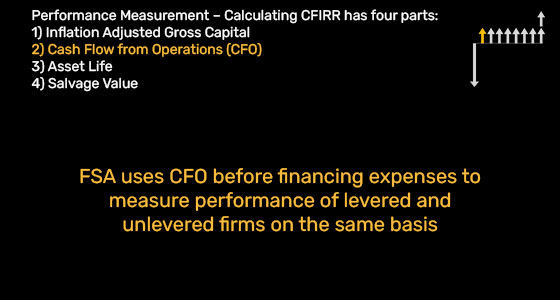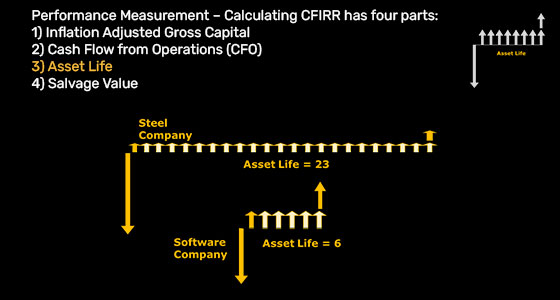## Asset Life

### Asset Life Can Affect the Rate of Return on Capital

The nature of some investments are long lasting while others are short-term The asset life can greatly affect the rate of return on capital.

For example, steel companies have much longer asset lives than software companies. CFIRR addresses the issue of asset life variance. This differs from traditional return on capital metrics that are ratios. Mathematically ratios are perpetuities which implies cash flows go on forever which is a very poor assumption and overstates the true economic return on capital.

Asset Life = (Gross Plant + Other Non-Current Assets  Inventories) / Depreciation Expense

## Salvage Value

### Adjustment for Cash on the Balance Sheet

Salvage value is the only adjustment CFIRR makes that improves the return on capital compared to traditional return on capital measures. It is an important adjustment for rapidly growing companies who often have large working capital balances just after large financings. By considering the working capital as salvage value, the CFIRR gives a more accurate calculation for return on deployed capital.

Salvage Value = Current Assets - Current Liabilities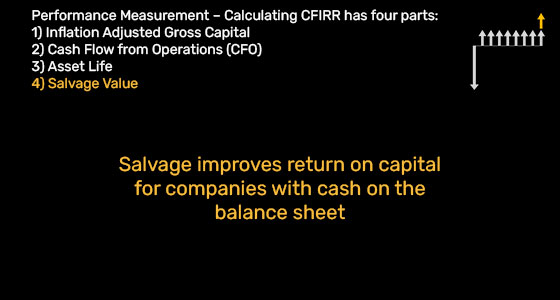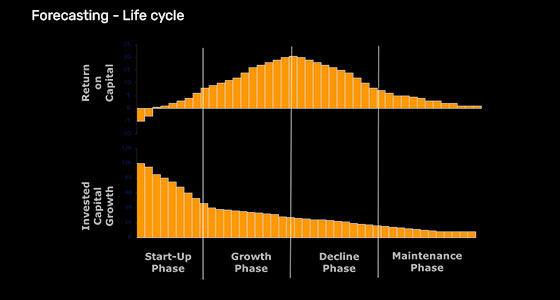## Forecasting

### Future Cash Flows are the Biggest Part of Valuation

Our valuation methodology accounts for all invested capital including all asset, liabilities and equity but also includes a forecast of future cash flows above the cost of capital.

In fact in most cases, the forecast is the largest component of the valuation. Unlike the balance sheet portion of our valuation whereby assets and liabilities are known with relative certainty (given high quality accounting and no impending write-offs), the forecasting portion of value involves the future and is therefore uncertain. However, we can model the future using the concept of a life cycle.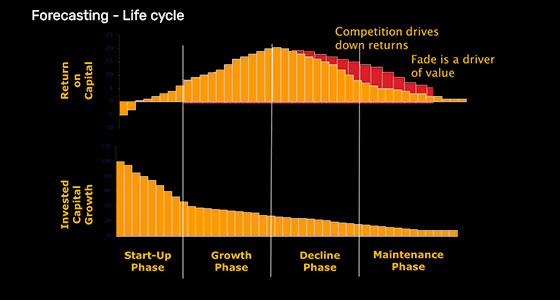## Forecasting

### Understand the “Fade” is Critical to Understanding Value

The degree to which a company can beat the fade adds tremendous value to the shareholders.

Beating the fade is a similar concept to monopoly rents which can occur via high barriers to entry, superior technology, strong branding, first mover advantage, etc.

Companies like Nike, Procter & Gamble, Amazon, Apple and Home Depot are all companies who are widely consider to have “beaten the fade”

## Valuation

### FSA Uses a Value Added Methodology

There are three steps to calculating the value of a stock  in our methodology

1. + Value of Net Invested Capital – known from the balance sheet
2. + Future Cash Flows – unknown
3. - Debt – known from the balance sheet
= Value of the Equity (i.e. shares)

The only unknown is Future Cash Flows.  We estimate the value of future cash flows as discussed in the forecasting section.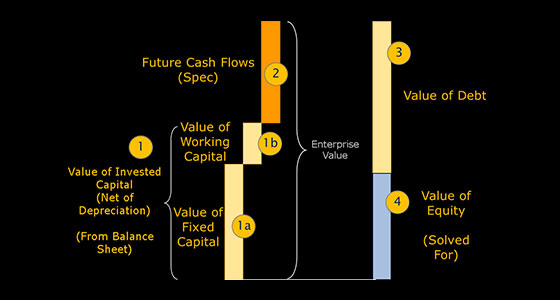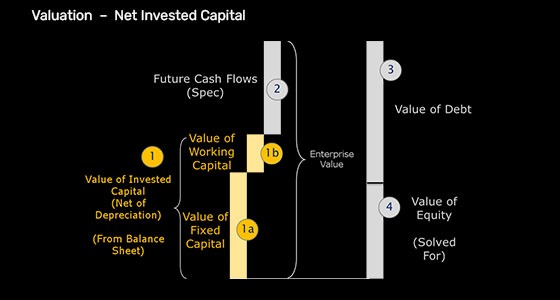## Value of Net Invested Capital

Recall that in performance measurement GROSS invested capital was the appropriate variable to use in calculating CFIRR. However in valuation, it is appropriate to use NET invested capital because we want to know how much the company is worth. If assets are old and depreciated we need to take this into account. The line items included here are the same as performance measurement for inflation adjusted gross capital with the exception that it is after accumulated depreciation.

## Future Cash Flows

This could also be called the "value added" and is the speculative portion of value.

If a forecast is a positive spread (i.e. CFIRR – Cost of Capital is positive), then value is created. In this case, the higher the capital growth forecast, the higher the valuation creation.

If a forecast is a negative spread (i.e. CFIRR – Cost of Capital is negative) then value is destroyed. In this case, the higher the capital growth forecast, the higher the value destruction.

If a forecast is a zero spread (i.e. CFIRR – Cost of Capital is zero), then growth does not affect value.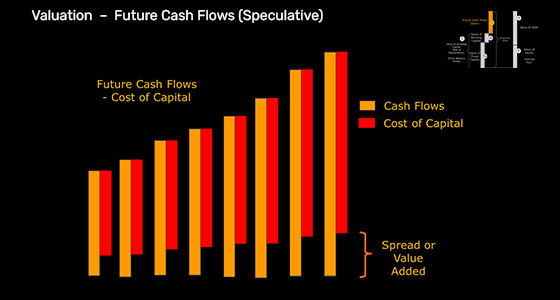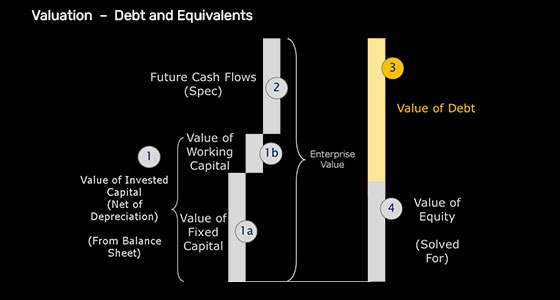## Valuation Of Debt

The value of debt (more accurately value of debt and equivalents) is calculated from the balance sheet as follows:
Value of Debt =
+ Long-Term Debt
+ Minority Interest (from Balance Sheet)
+ Other Non-Current Liabilities (including Pension liabilities)
+ Preferred Stock
+ Short-Term Debt
Other items that could be added to value of debt would be off balance sheet liabilities such as pending lawsuits and under-funded pensions.

## Value of Equity

All the above calculations lead to the value of the equity. The CFIRR and capital growth history lead to the CFIRR and capital growth forecasts, including the fade. The forecast produces a stream of cash flows less the cost of capital that is discounted to the present value. These discounted cash flows are added to the value of net invested capital to produce the total enterprise value. Finally, the value of equity is calculated as the total enterprise value less the value of debt.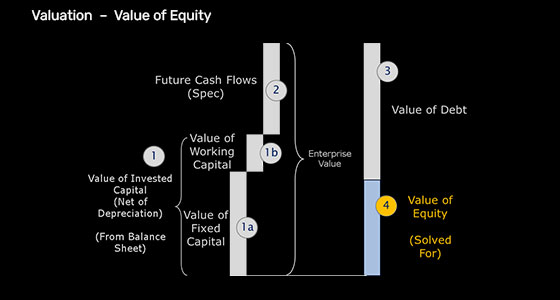## FSA Valuation Service

220 Victoria Street
Suite 2006

Toronto, ON M5B 2R6

info@fsavaluation.com